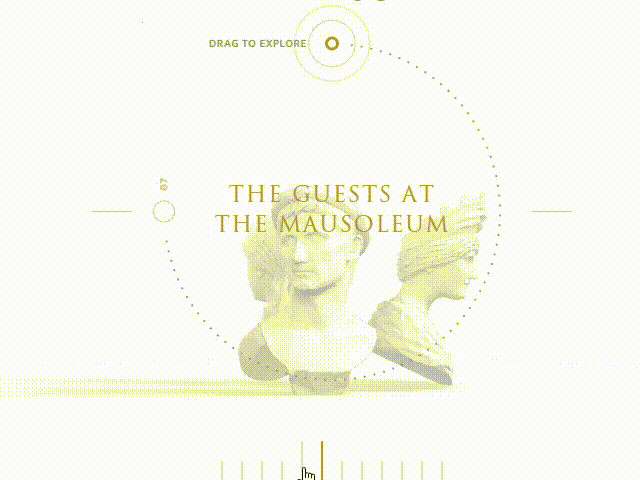# Morph geometry tool

Hola, I want to achieve a modern effect when two lowpoly models morph in each other by triangles.

Like this guysSo, I see next steps:

1. Find out first model’s geometry;
2. Find out target model’s geometry.
3. Build a lot of triangles in source model’s geometry
4. Move, rotate and scale em one by one to new place
5. ???
6. Profit!

First four steps are most difficult and I do need some advices.

So I don’t know how to get actual information about model’s geometry.
Is there a way to get all triangles of the mesh?

Thanks a lot.

I would do this with a vertex shader and a per-face vertex color. The vertex shader has a uniform that moves the vertex by a direction defined by the vertex color. Then, by setting this uniform you can make the model ‘explode’ as in the image.

It will also allow you to set how exploded with the uniform. This means you can have two models - one that explodes, and one that un-explodes. Played simultaneously, it will have this effect. No morphing (which is hard) required.

In fact, I think the above gif uses this technique. You will notice that in between morphing, there appear to be many more particles than in either model - there’s a sudden flurry of them. I suspect this is when both the incoming and outgoing model are visible.